# The Kontsevich Integral for Knotted Trivalent Graphs

In Preparation

The information below is preliminary and cannot be trusted! (v)

## Abstract

We construct a (very) well-behaved invariant of knotted trivalent graphs using only the Kontsevich integral, in three steps.

## Step 1 - The Naive Kontsevich Integral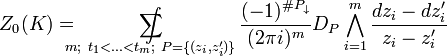$Z_0(K)=\ \ \ \ \ \ \ \ \ \ \int\!\!\!\!\!\!\!\!\!\!\!\!\!\!\!\!\!\!\!\!\!\!\!\!\!\!\!\!\!\!\sum_{m;\ t_1<\ldots

We define the "naive Kontsevich integral"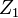$Z_1$ of a knotted trivalent graph or a slice thereof as in the "standard" picture above, except generalized to graphs in the obvious manner.

### The Good

• It has a factorization property.
• For the "braid-like" factors, it has invariance under horizontal deformations.
• It is morally a universal finite type invariant.

• It is divergent.

## Step 2 - The Renormalized Kontsevich Integral

After choosing a scale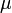$\mu$ and an infinitesimal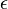$\epsilon$ and using ideas from renormalization theory as practiced by quantum field theorists, we define the "renormalized Kontsevich integral"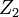$Z_2$ of a knotted trivalent graph or a slice thereof as summarized by the picture above.

### The Good

• It retains all the good properties of the naive Kontsevich integral.
• It is convergent.
• It is invariant under rigid motions of critical points and trivalent vertices.
• It has sensible behaviour under vertical connect sum, delete and unzip operations.
• It has a sensible behaviour under the changing of the scale$\mu$, as follows from:

Claim. Let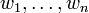$w_1,\ldots,w_n$ be distinct complex numbers and let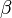$\beta$ be another complex number. Let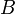$B$ be the ($n$-strand) braid "rescaling braid" which is the image of the map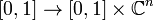$[0,1]\to[0,1]\times{\mathbb C}^n$ defined by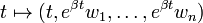$t\mapsto(t, e^{\beta t}w_1,\ldots,e^{\beta t}w_n)$. Finally, in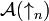${\mathcal A}(\uparrow_n)$ let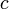$c$ be the "sum of all horizontal chords";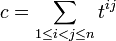$c=\sum_{1\leq i. Then up to normalization factors which we are getting right with probability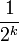$\frac{1}{2^k}$ for some small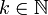$k\in{\mathbb N}$,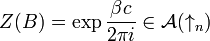$Z(B)=\exp\frac{\beta c}{2\pi i}\in{\mathcal A}(\uparrow_n)$.

By correcting caps and cups with factors$n$ and$u$, and the two types of trivalent vertices with factors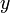$y$ and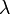$\lambda$, we define the "corrected Kontsevich integral"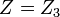$Z=Z_3$ of a knotted trivalent graph or a slice thereof. It has all the good properties we can wish for. The details still need to be worked out here!
The Drinfel'd KZ associator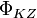$\Phi_{KZ}$ is reproduced already by$Z_2$: